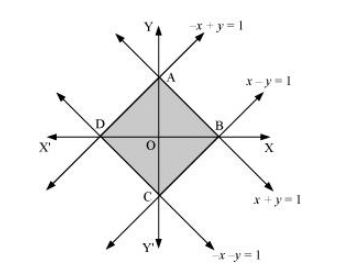# Using the method of integration find the area bounded by the curve

Question:

Using the method of integration find the area bounded by the curve $|x|+|y|=1$

[Hint: the required region is bounded by lines $x+y=1, x-y=1,-x+y=1$ and $-x-y=11$ ]

Solution:

The area bounded by the curve, $|x|+|y|=1$, is represented by the shaded region ADCB asThe curve intersects the axes at points A (0, 1), B (1, 0), C (0, –1), and D (–1, 0).

It can be observed that the given curve is symmetrical about x-axis and y-axis.

∴ Area ADCB = 4 × Area OBAO

$=4 \int_{0}^{1}(1-x) d x$

$=4\left(x-\frac{x^{2}}{2}\right)_{0}^{1}$

$=4\left[1-\frac{1}{2}\right]$

$=4\left(\frac{1}{2}\right)$

$=2$ units